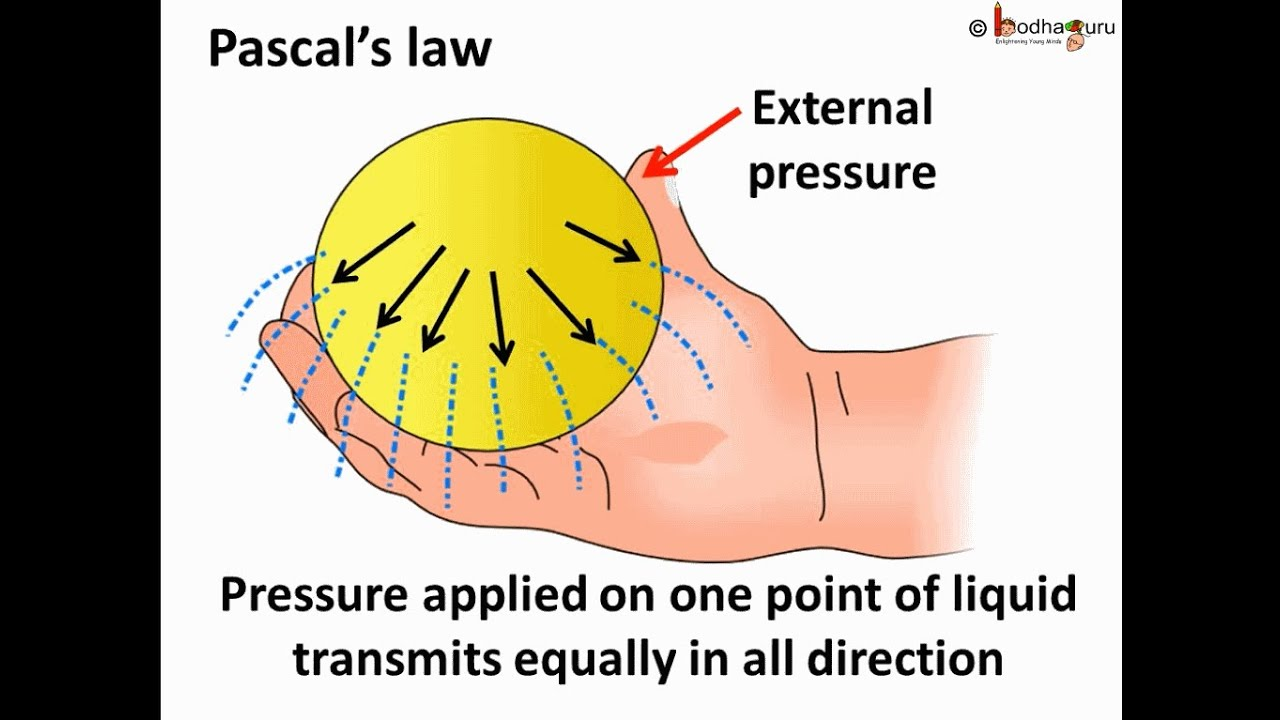## Pascal's Law

Subject: Science

#### Overview

Pascal's law states that "Pressure exerted by liquid in an enclose vessel is transmitted equally". This law is applicable for the gases also. This is the detailed note on pascal's law and its application in hydraulic press.
##### Pascal's Law

Pascal's law states that "Pressure exerted by liquid in an enclose vessel is transmitted equally". This law was formulated by Blaise Pascal who was born in 1623 in France. This law is applicable for the gases also. The verification of Pascal's law can be done with this simple experiment:Take a plastic (or a balloon) and poke small holes (carefully) in the plastic. Fill the plastic with water and seal the top big opening. Slowly press the plastic from the top and watch how water flows through each hole. Water should flow equally from all the pores because the pressure applied from the top is distributed equally to all the holes.

#### Hydraulic press

This is an equipment based on Pascal's law. To create hydraulic press we need two or more pistons, of different cross section area, along with water or oil. A structure is made as shown in the picture and liquid is added into it. Then the pistons of matching size are inserted in the opening and the openings are sealed. The openings are sealed in such a way that the pistons are allowed to move freely pressing the liquid. When small piston is pressed the pressure is distributed equally in the liquid. So the larger piston will move upward. According to the Pascal's law, pressure in the small piston is equal to the pressure on the larger piston.i.e. P1 = P2

or,=or, F2 =or, F2 = F1 x ratio of larger piston to smaller piston.

This shows more the area of the ratio of larger piston to smaller piston the force will be bigger. The same law is applied in hydraulic brakes and hydraulic lifts.

##### Things to remember
• Pascal's law states that "Pressure exerted by liquid in an enclose vessel is transmitted equally".
• Pascal's law was formulated by Blaise Pascal who was born in 1623 in France.
• Hydraulic press is an equipment based on Pascal's law.
• It includes every relationship which established among the people.
• There can be more than one community in a society. Community smaller than society.
• It is a network of social relationships which cannot see or touched.
• common interests and common objectives are not necessary for society.
##### Videos for Pascal's Law
Pascal's law states that, "The pressure is equally exerted perpendicularly on all sides as pressure is applied at a place on a liquid contaied in a closed container".
Hydraulic press, Hydraulic break and hydraulic lift are three examples that uses pascal's law.
Automobiles use hydraulic breaking system. When driver applies small force into the brake pad it has applied in a small area but when that force reaches to the break pad of the truck (which has large area) the force is magnified to the original times the area of the break pad, according to pascal's law. So a heavy loaded truck be stopped by appling a small force by a driver.
Upthrust is the upward force exerted by liquid acting on an immersed body. It is the resultant thrust acting upward by the liquid.
Density of a substance is defined as the mass per unit volume.
The relative density of a body is defined as the ratio of the mass of a particular volume of that body to the mass of same volume of water at 4 degree centigrade. It is aldo called relative density.
The force that acts perpendicular to and into a surface is called thrust.

Pascal's law states that liquid exerts pressure equally in all directions.

Given,

Depth of water (h) = 6m

Density of water (d) = 1000 kg/m3

Acceleration due to gravity(g) =9.8 m/sec2

Pressure of water (P) =?

We know,

P= h x d x g

= 6 x 1000 x 9.8

= 58800 Pa

Hence, the water pressure exerted at the bottem of the tank is 58800 Pascal.